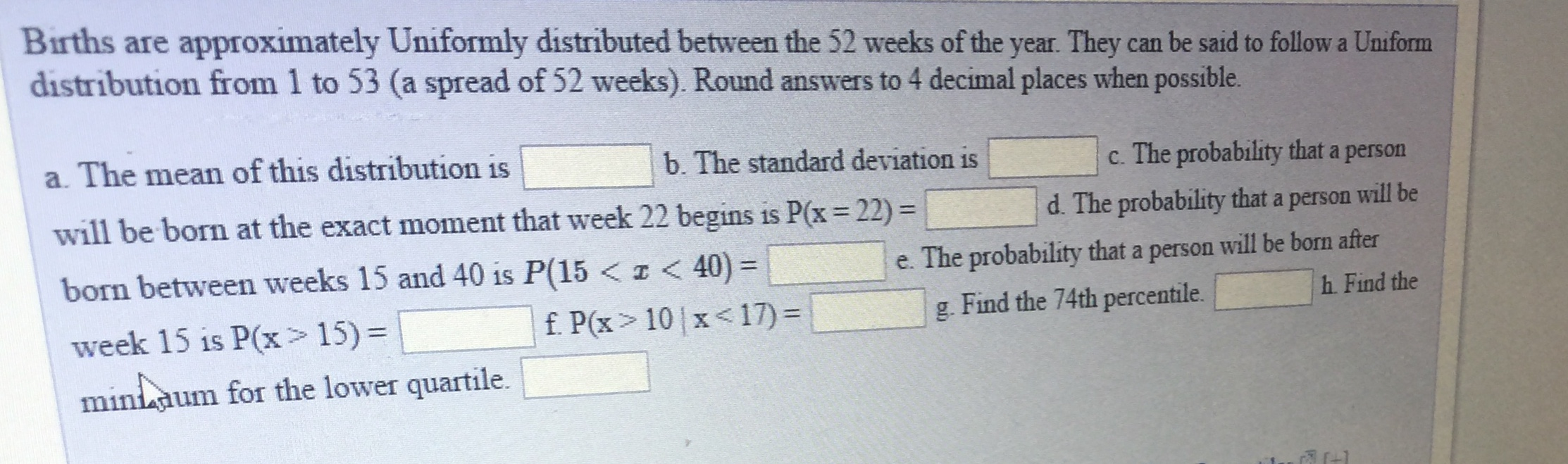Births are approximately Uniformly distributed between the 52 weeks of the year. They can be said to follow a Uniformdistribution from 1 to 53 (a spread of 52 weeks). Round answers to 4 decimal places when possible.a. The mean of this distribution iswill be born at the exact moment that week 22 begins is Px 22)born between weeks 15 and 40 is P(15 10 1 x

Questionhelp_outlineImage TranscriptioncloseBirths are approximately Uniformly distributed between the 52 weeks of the year. They can be said to follow a Uniform distribution from 1 to 53 (a spread of 52 weeks). Round answers to 4 decimal places when possible. a. The mean of this distribution is will be born at the exact moment that week 22 begins is Px 22) born between weeks 15 and 40 is P(15 10 1 x<17) g. Find the 74th percentile. h. Find the um for the lower quartile. fullscreen
Step 1

Hi, This question has multiple parts and in such cases we are required to answer just the first three parts. If you need answers to other parts, repost mentioning exactly which parts you need answered.

Mean of a uniform distribution with intervals a and b is given by:

Mean = (a+b)/2

Standard Deviation of a uniform disribution with intervals a and b is given by

Standard deviation = (b-a)/ (12)^0.5

Here, a= 1 and b = 53

therefore,

Mean = 1 + 53 / 2 = 27

Standard Deviation = 15.011107 ~ 15.0111

Step 2

In any continuous distribution, the probability of any variable to be an exact number is always equal to 0.

Mathematically, P(X= n) = 0   always ( where n is an exact number or point)

Probability of a variable to be less than a number or to be more ...

Want to see the full answer?

See Solution

Want to see this answer and more?

Our solutions are written by experts, many with advanced degrees, and available 24/7

See Solution
Tagged in

Hypothesis Testing